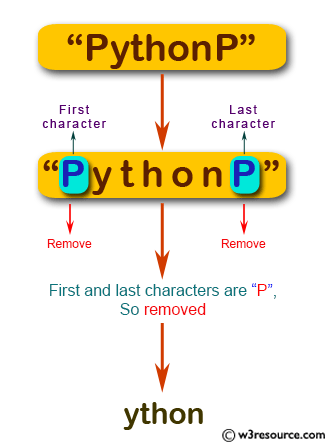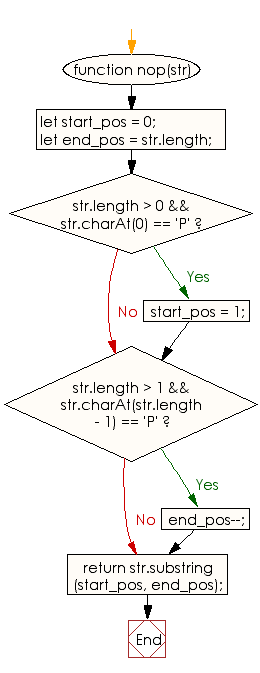# JavaScript: Create a new string from a given string, removing the first and last characters of the string if the first or last character are 'P'

## JavaScript Basic: Exercise-67 with Solution

Write a JavaScript program to create a new string from a given string, removing the first and last characters of the string if the first or last character are 'P'. Return the original string if the condition is not satisfied.

Pictorial Presentation:Sample Solution:

HTML Code:

``````<!DOCTYPE html>
<html>
<meta charset="utf-8">
<meta name="viewport" content="width=device-width">
<title>JavaScript program to create a new string from a given string, removing the first and last characters of the string if the first or  last character are �P</title>
<body>

</body>
</html>
```
```

JavaScript Code:

``````function nop(str) {
let start_pos = 0;
let end_pos = str.length;

if (str.length > 0 && str.charAt(0) == 'P')
{
start_pos = 1;
}

if (str.length > 1 && str.charAt(str.length - 1) == 'P')
{
end_pos--;
}

return str.substring(start_pos, end_pos);
}

console.log(nop("PythonP"));
console.log(nop("Python"));
console.log(nop("JavaScript"));
```
```

Sample Output:

```ython
ython
JavaScript
```

Flowchart:ES6 Version:

``````function nop(str) {
let start_pos = 0;
let end_pos = str.length;

if (str.length > 0 && str.charAt(0) == 'P')
{
start_pos = 1;
}

if (str.length > 1 && str.charAt(str.length - 1) == 'P')
{
end_pos--;
}

return str.substring(start_pos, end_pos);
}

console.log(nop("PythonP"));
console.log(nop("Python"));
console.log(nop("JavaScript"));
``````

Live Demo:

What is the difficulty level of this exercise?

Test your Programming skills with w3resource's quiz.

﻿

## JavaScript: Tips of the Day

Checks if a string is an anagram of another string (case-insensitive, ignores spaces, punctuation and special characters)

Example:

```const isAnagram = (str1, str2) => {
const normalize = str =>
str
.toLowerCase()
.replace(/[^a-z0-9]/gi, '')
.split('')
.sort()
.join('');
return normalize(str1) === normalize(str2);
};
console.log(isAnagram('iceman', 'cinema')); // true
```

Output:

```true
```